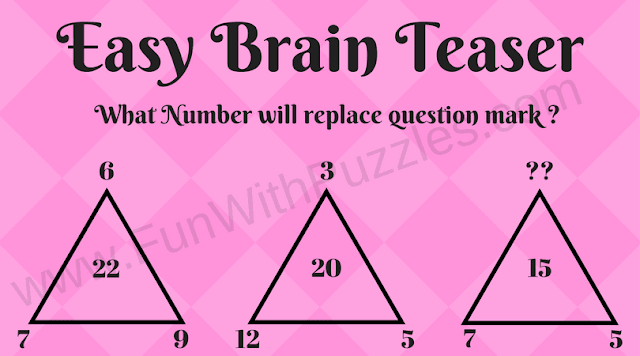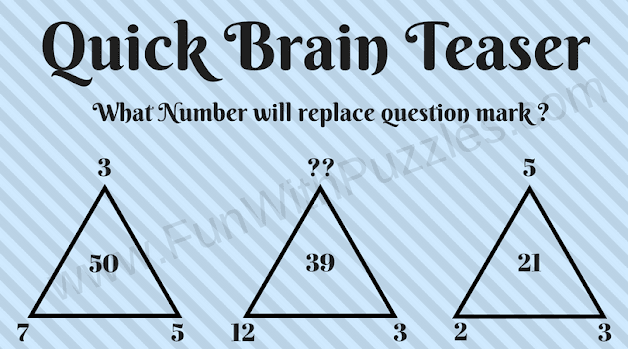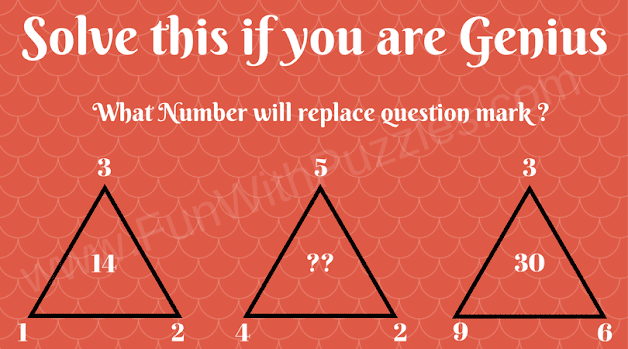Mathematics is a very interesting subject. However, it depends upon the way it is kids learn mathematics. If we interestingly teach kids math, they will love mathematics. Solving puzzles is a very fun way to learn Mathematics. One can have fun solving Mathematical Brain Teasers and also learn the basics of Maths. Here are some brain teasers which can be used by school-going kids and teens to learn the basics of mathematics.

## How to Solve Triangle Puzzles

In each of the Triangle Math Brain Teasers, three triangles are given. Each corner of the triangle, as well as the center of the triangle, contains a number. One of the numbers in one of the triangles is replaced with the question mark.  These numbers have some relationship with each other. One has to find this relationship and find the number which will replace the triangle. So are you ready to find which number replaces the question mark in each triangle?1. Find the Missing Number in the 3rd Triangle?2. Which Number will replace the question mark?3. Can you find the missing number?4. Test Your Brain by Finding the Missing Number5. Which number will replace the question mark?

Finding the missing number is a very interesting Maths Picture puzzle. Check out our similar below-listed Maths Missing Number Picture Puzzles to challenge your brain

## List of Missing Number Picture Puzzles

1. Awesome Missing Number Math Circle Puzzles with Answers: These are Maths Circle Picture Puzzles in which your challenge is finding the missing number in the Circle.

2. Mind-Blowing Maths Circle Questions with answers: This is another set of Maths Circle Missing Number Puzzles. However, these are tough puzzles and will twist your brain.

3. Test Your Brain-Smart Questions For Smart People: It contains a mix of Maths Puzzles, Visual Observational Puzzles, and Spatial Puzzles to test your brain.

4. Brain Maths Logic Puzzles for Teens: At the end, this set contains the Brain Teasers in which you have to use your Logical Reasoning as well as Mathematical skills.